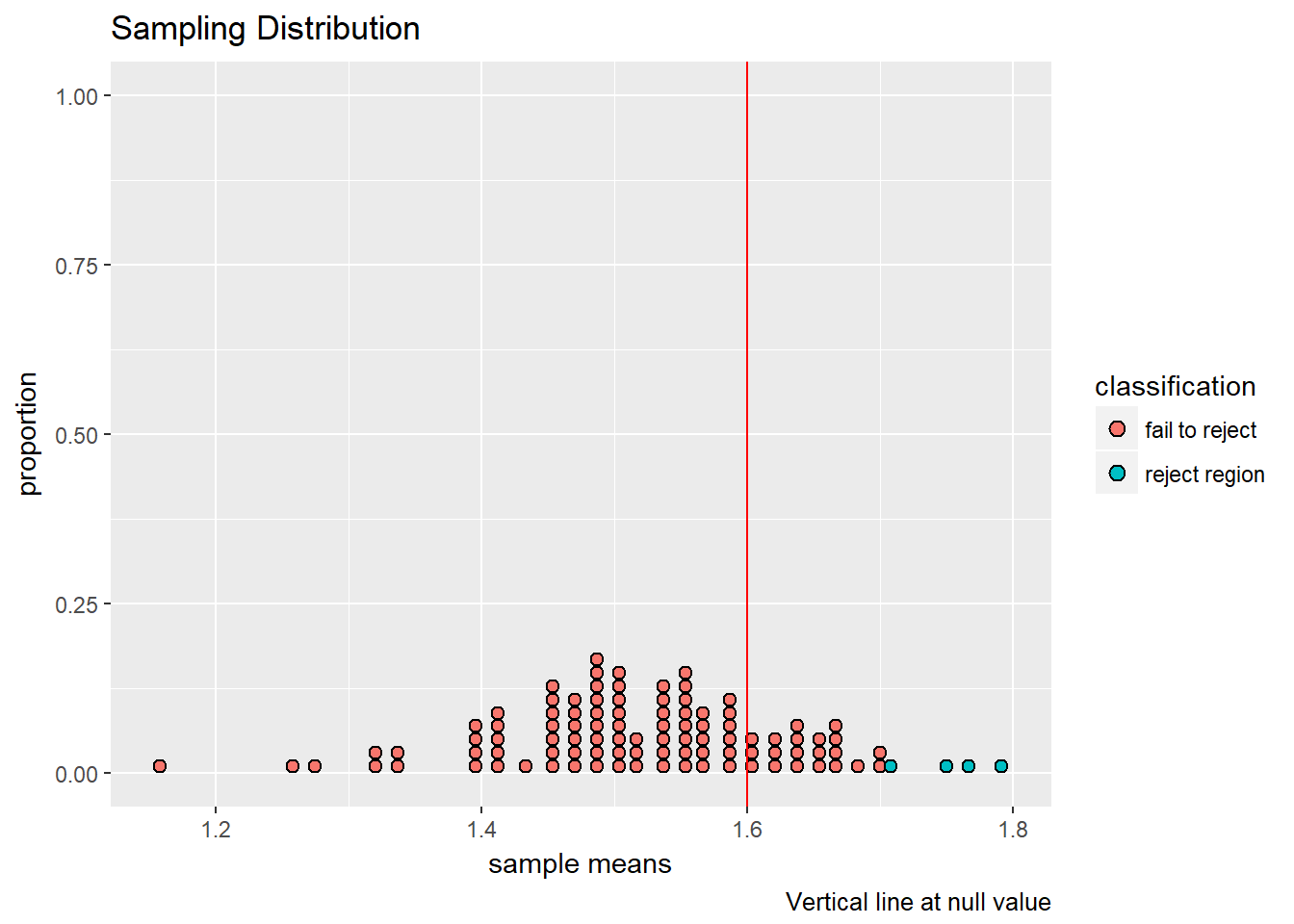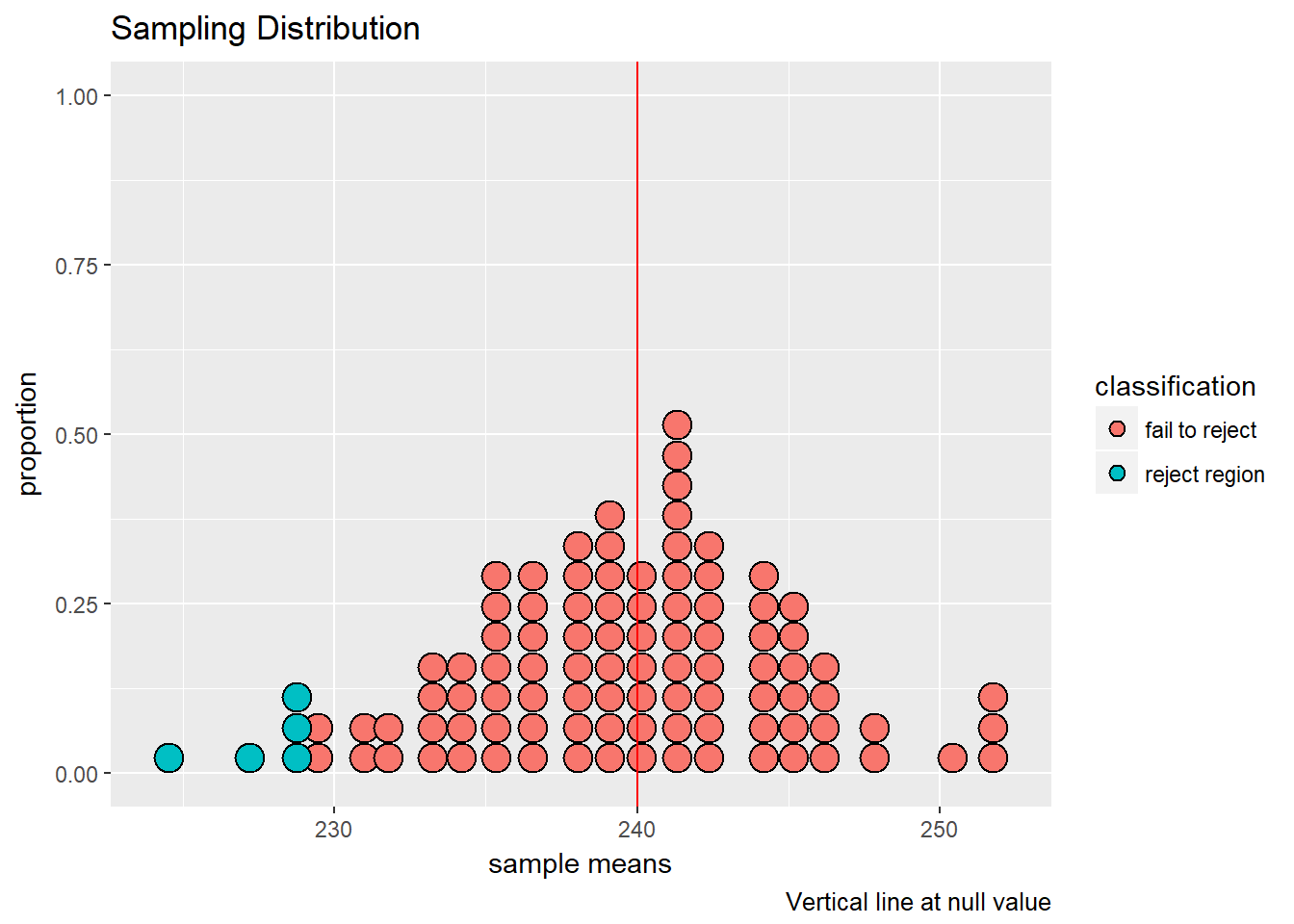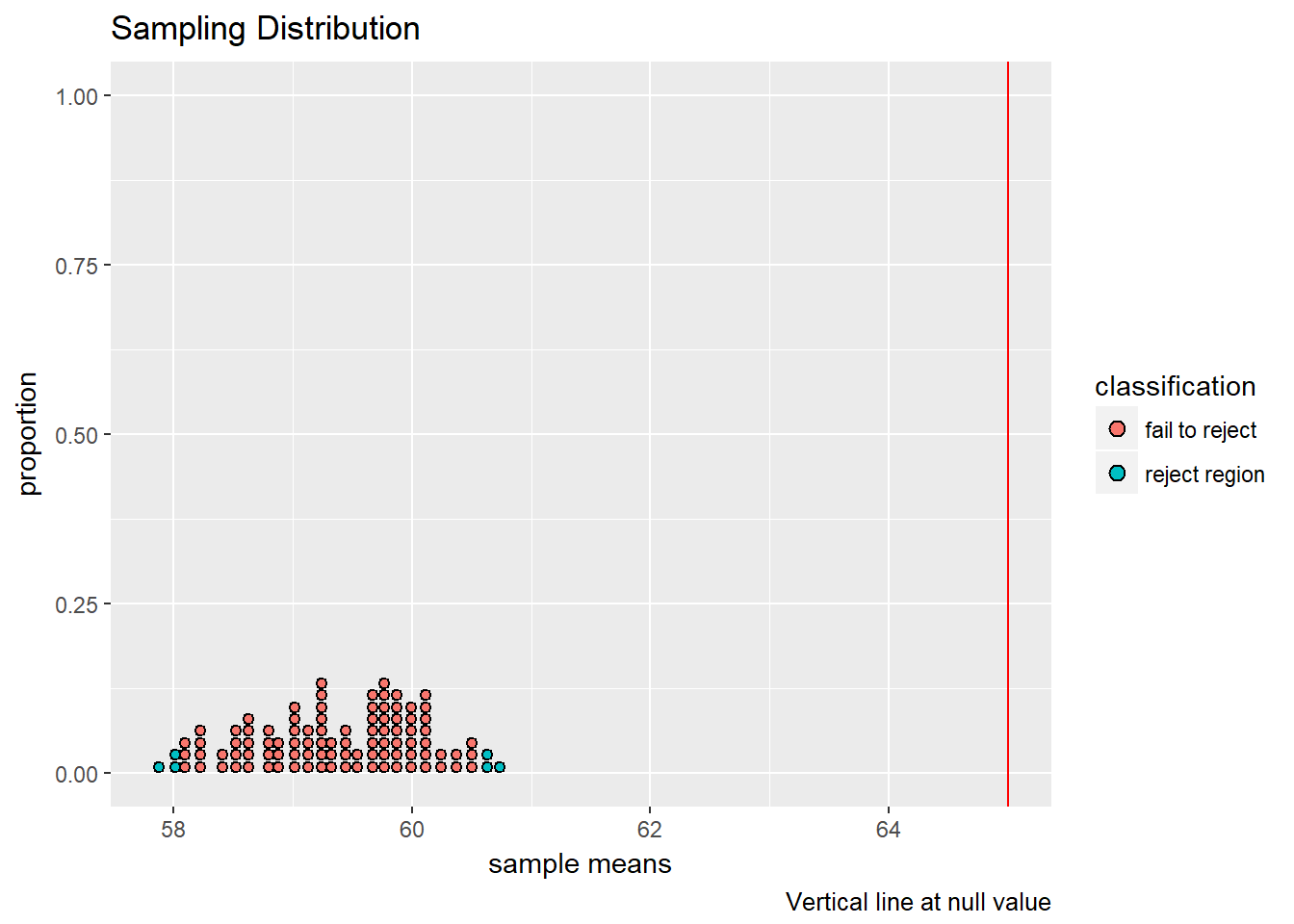The data comes from the UCI Machine Learning Repository, and this specific data set describes patients at the VA hospital in Long Beach, California (for more detailed information, go to this data set description).

Use the following code to load the data. An internet connection is required. Install the packages beforehand if need be.

library("tidyverse") #enables tbl_df() function

col_attributes <- c("age", "sex", "cp",
"trestbps", "chol", "fbs",
"restecg", "thalach", "exang",
"oldpeak", "slope", "ca",
"thal", "num") # used later to rename the columns

# heart_df <- read_csv("http://archive.ics.uci.edu/ml/machine-learning-databases/heart-disease/processed.va.data", col_names = col_attributes)
heart_df <- read_csv("http://mlr.cs.umass.edu/ml/machine-learning-databases/heart-disease/processed.va.data", col_names = col_attributes) #use this link if the UCI website is down
heart_df <- tbl_df(heart_df)
heart_df$chol[heart_df$chol < 100] <- NA #re-labels missing values to "NA"
heart_df
## # A tibble: 200 x 14
##      age   sex    cp trestbps  chol   fbs restecg thalach exang oldpeak
##    <int> <int> <int>    <chr> <chr> <chr>   <int>   <chr> <chr>   <chr>
##  1    63     1     4      140   260     0       1     112     1       3
##  2    44     1     4      130   209     0       1     127     0       0
##  3    60     1     4      132   218     0       1     140     1     1.5
##  4    55     1     4      142   228     0       1     149     1     2.5
##  5    66     1     3      110   213     1       2      99     1     1.3
##  6    66     1     3      120  <NA>     0       1     120     0    -0.5
##  7    65     1     4      150   236     1       1     105     1       0
##  8    60     1     3      180  <NA>     0       1     140     1     1.5
##  9    60     1     3      120  <NA>     ?       0     141     1       2
## 10    60     1     2      160   267     1       1     157     0     0.5
## # ... with 190 more rows, and 4 more variables: slope <chr>, ca <chr>,
## #   thal <chr>, num <int>

## One-Sided Hypothesis Testing

The num column in the data frame refers to risk of a heart attack, with 0 = “no risk” and 4 = “high risk”. Here we will test the claim that the average risk level is 1.6 with the following setup with the null and alternative hypothesis respectively: $H_{0}: \mu = 1.6$ $H_{a}: \mu > 1.6$

• Define $$n$$ as the number of patients in the data set and set the null value.
n <- nrow(heart_df)
null_value <- 1.6
• Use the following code to simulate $$N = 100$$ samples and compute their means. It is common practice in machine learning to make training sets with about 60 percent of the observations.
sample_means <- replicate(100, mean(sample(heart_df$num, n*0.60, replace = TRUE))) df <- data.frame(sample_means, null_value) • Define a threshold at the 95th percentile, and create a classification column that labels results in the sampling distribution that are above the threshold as being in the “reject region” (“fail to reject” otherwise). threshold <- quantile(sample_means, 0.95) df <- df %>% mutate(classification = ifelse(sample_means > threshold, "reject region", "fail to reject")) • Finally, use the following code to graph the results. ggplot(df, aes(x = sample_means, fill = classification)) + geom_dotplot(binwidth = 0.01) + geom_vline(aes(xintercept = null_value), col = "red") + labs(caption = "Vertical line at null value", title = "Sampling Distribution", x = "sample means", y = "proportion")The p-value is the probability of a false null hypothesis. Here we compute the p-value empirically as the proportion of sample means that are less than the null value. p_value <- mean(sample_means <= null_value) p_value ##  0.78 In general, to perform these simulations quickly and thoroughly in R, we use the t.test command. t.test(heart_df$num, mu = 1.6, alternative = "greater")
##
##  One Sample t-test
##
## data:  heart_df$num ## t = -0.92778, df = 199, p-value = 0.8227 ## alternative hypothesis: true mean is greater than 1.6 ## 95 percent confidence interval: ## 1.377505 Inf ## sample estimates: ## mean of x ## 1.52 Visually, and since the p-value 0.8226768 > 0.05, we fail to reject the claim at the $$\alpha = 0.05$$ significance level. A total cholesterol level less than 240 milligrams is desirable for adults (source). Test the claim that these patients are healthy with the following setup: $H_{0}: \mu = 240$ $H_{a}: \mu < 240$ #converts characters to numbers heart_df$chol <- as.numeric(heart_df$chol) • Set the null value. null_value <- 240 • Use the following code to simulate $$N = 100$$ samples and compute their means. It is common practice in machine learning to make training sets with about 60 percent of the observations. sample_means <- replicate(100, mean(sample(heart_df$chol, n*0.60, replace = TRUE),
na.rm = TRUE))
df <- data.frame(sample_means, null_value)
• Define a threshold at the 5th percentile, and create a classification column that labels results in the sampling distribution that are below the threshold as being in the “reject region” (“fail to reject” otherwise).
threshold <- quantile(sample_means, 0.05)
df <- df %>%
mutate(classification = ifelse(sample_means < threshold, "reject region", "fail to reject"))
• Finally, use the following code to graph the results.
ggplot(df, aes(x = sample_means, fill = classification)) +
geom_dotplot(binwidth = 1) +
geom_vline(aes(xintercept = null_value), col = "red") +
labs(caption = "Vertical line at null value",
title = "Sampling Distribution",
x = "sample means",
y = "proportion")The p-value is the probability of a false null hypothesis. Here we compute the p-value empirically as the proportion of sample means that are less than the null value.

p_value <- mean(sample_means >= null_value)
p_value
##  0.48

In general, to perform these simulations quickly and thoroughly in R, we use the t.test command.

t.test(heart_df$chol, mu = null_value, alternative = "less") ## ## One Sample t-test ## ## data: heart_df$chol
## t = -0.097874, df = 143, p-value = 0.4611
## alternative hypothesis: true mean is less than 240
## 95 percent confidence interval:
##      -Inf 246.8524
## sample estimates:
## mean of x
##  239.5694

Visually, and since the p-value 1 > 0.05, we fail to reject the claim at the $$\alpha = 0.05$$ significance level.

## Two-Sided Hypothesis Testing

Test the claim that heart-risk patients are elderly with the following setup: $H_{0}: \mu = 65$ $H_{a}: \mu \neq 65$

• Set the null value.
null_value <- 65
• Use the following code to simulate $$N = 100$$ samples and compute their means. It is common practice in machine learning to make training sets with about 60 percent of the observations.
sample_means <- replicate(100,
mean(sample(heart_df$age, n*0.60, replace = TRUE), na.rm = TRUE)) df <- data.frame(sample_means, null_value) • Define thresholds at the 2.5 and 97.5 percentiles, and create a classification column that labels results in the sampling distribution that are outside the thresholds as being in the “reject region” (“fail to reject” otherwise). left_threshold <- quantile(sample_means, 0.025) right_threshold <- quantile(sample_means, 0.975) df <- df %>% mutate(classification = ifelse(sample_means < left_threshold | sample_means > right_threshold, "reject region", "fail to reject")) • Finally, use the following code to graph the results. ggplot(df, aes(x = sample_means, fill = classification)) + geom_dotplot(binwidth = 0.1) + geom_vline(aes(xintercept = null_value), col = "red") + labs(caption = "Vertical line at null value", title = "Sampling Distribution", x = "sample means", y = "proportion")The p-value is the probability of a false null hypothesis. Here we compute the p-value empirically as the proportion of sample means that are less than the null value. p_value <- mean(2*sample_means <= null_value) p_value ##  0 In general, to perform these simulations quickly and thoroughly in R, we use the t.test command. t.test(heart_df$age, mu = null_value, alternative = "two.sided")
##
##  One Sample t-test
##
## data:  heart_df\$age
## t = -10.229, df = 199, p-value < 2.2e-16
## alternative hypothesis: true mean is not equal to 65
## 95 percent confidence interval:
##  58.26075 60.43925
## sample estimates:
## mean of x
##     59.35

Visually, and since the p-value 5.279306710^{-20} > 0.05, we reject the claim at the $$\alpha = 0.05$$ significance level.